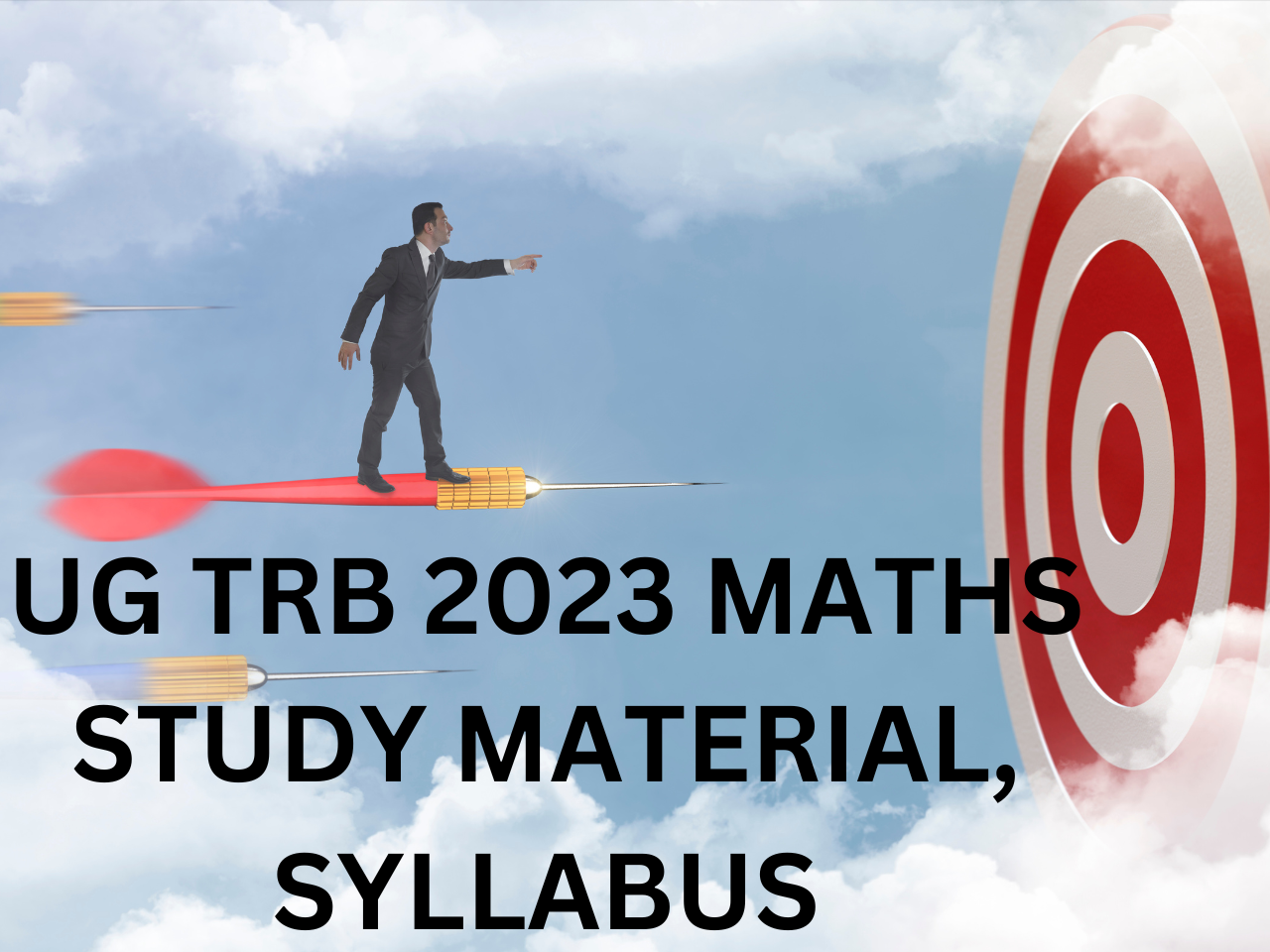# KARKANDU KANITHAM# UG TRB 2023 MATHS | STUDY MATERIAL, SYLLABUS

## UG TRB 2024 MATHS STUDY MATERIAL:

UNIT – 1: ALGEBRA:

UNIT-1 ALGEBRA and TRIGONOMETRY

Polynomial Equations – Imaginary and Irrational Roots – Relation between Roots and Coefficients symmetric function of Roots in terms of coefficient- Transformation of equation – Reciprocal equation – Increase or Decrease the roots of given equation – Removal of terms – Descartes’s rule of signs – Approximate solution of roots of polynomial by Horner’s Method–Cardan’s method of solution of cubic polynomial – Summation of series using Binomial – Exponential and Logarithmic series.

Symmetric – Skew symmetric, Hermitian – Skew Hermitian, Orthogonal Matrices, Unitary Matrices – Eigen Values – Eigen Vectors – Cayley-Hamilton Theorem – Similar Matrices – Diagonalization of Matrices.

Prime Number, Composite Number, Decomposition of a Composite Number as a Product of primes uniquely – Divisor of a positive Integer – Euler Function. Congruence Modulo n, Highest power of prime number p Contained in n! – Application of Maxima and Minima – Prime and Composite numbers – Euler’s function 􀝊(N) – Congruences – Fermat’s, Wilson’s and Lagrange’s theorems.

Expansions of Power of sinnX, cosnX, tannx – Summation by C + i S method, Telescopic Summation – Expansion of sinx, cosx, tanx in terms of x – Sum of Roots of Trigonometric Equation, Formation of Equation With Trigonometric Roots – Hyperbolic Functions – Relation Between Circular and Hyperbolic Function – Inverse Hyperbolic Function – Logarithm of a complex number – Principal Value and General Values.

UG TRB 2024 MATHS STUDY MATERIALS ARE AVAILABLE NOW.

FOR DETAILS CONTACT: 9486136884